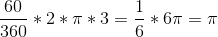## Example Questions

### Example Question #6 : How To Find The Distance Between Clock Hands

A clock has two equally long hands on it, each measuringinches. If the minute hand is directly onand the hour hand is directly on, what is the distance between the two hands?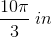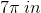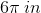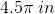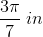Explanation:

Our clock looks roughly like this: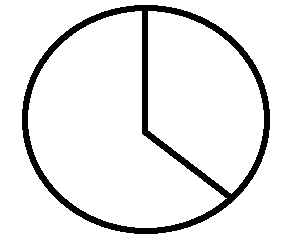Now, between every number on the clock, there are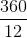ordegrees. Therefore, fromto, there are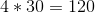degrees. To find the arc length, you use the equation: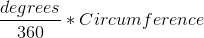Now, we know:We know that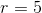. Therefore, we can write our equation: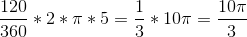### Example Question #8 : How To Find The Distance Between Clock Hands

A clock has two equally long hands on it, each measuringinches. If the minute hand is directly onand the hour hand is directly in the middle ofand, what is the distance between the two hands?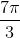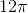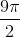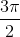Explanation:

Our clock looks roughly like this: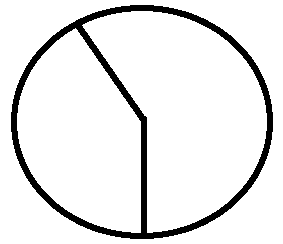Now, between every number on the clock, there areordegrees. We have a little trickier math to do, however. Let's subdivide the clock intosubsections instead. Each of these will havedegrees. Now, betweenand, there aresuch subsections. Since the hour hand is directly in the middle ofand, there is one more such subsection. Therefore, we havetotal subsections or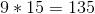degrees. To find the arc length, you use the equation:Now, we know:We know that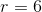.  Therefore, we can write our equation: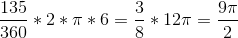### Example Question #7 : How To Find The Distance Between Clock Hands

A clock has two equally long hands on it, each measuringinches and extending to the edge of the clock. If the minute hand is directly onand the hour hand is directly on, what is the length of the minor arc connecting the two hands?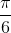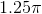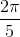Explanation:

Our clock looks roughly like this: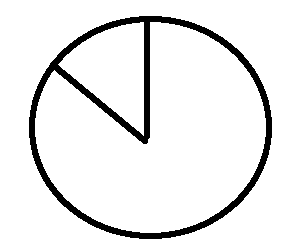Now, between every number on the clock, there areordegrees. Therefore, fromtothere areof these sectors. Therefore, there are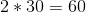degrees. To find the arc length, you use the equation:Now, we know:We know that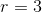. Therefore, we can write our equation: